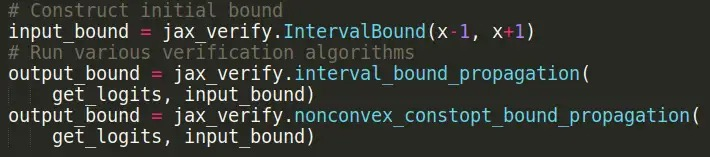We present recent developments in neural network verification and a library in JAX implementing these along with several other neural network verification methods.

Neural network verification is a powerful technology, offering the promise of provable guarantees on networks satisfying desirable input-output properties or specifications. Much progress has been made on neural network verification, focused on incomplete verifiers, i.e., verification algorithms that guarantee that the property is true if they return successfully, but may fail to verify properties that are true. Figure A shows how incomplete verifiers work for a simple feedforward network on MNIST. We are interested in checking if small perturbations of the input image of a 9 can lead to a misclassification. Geometrically, the space of perturbations is represented by the pink region in the lower panel and incomplete verifiers work by propagating over-approximations or relaxations of the set of activations realisable at a given layer under input perturbations - the exact regions are shown in pink and the relaxations in green. If the green region at the output layer does not intersect the specification (i.e. the hyperplane separating region of outputs that satisfies the constraint that the most likely label remains a 9 from the rest), then we have successfully verified the specification.

In these verification algorithms, there is often a trade-off between the computational efficiency of the verification algorithm and its “tightness”, i.e. the gap between properties that are known to be true and properties that the verification method is able to verify to be true (see Figure B).

We have recently developed new verification algorithms that seek to improve this trade-off, enabling both tight and computationally efficient verification algorithms:

Efficient nonconvex reformulations of neural network convex relaxations [Hinder et al, NeurIPS 2020]: Verification algorithms for neural networks are often derived from a convex relaxation, that replaces the nonlinear relations between network activations by a weaker set of convex (often linear) constraints between inputs and outputs of neurons (Ehlers 2017). This enables incomplete verification via convex optimisation, with tightness governed by the gap between the weaker convex and the original nonlinear constraints. However, off-the-shelf convex optimisation solvers still don’t scale efficiently to modern neural networks, and most attempts at developing scalable methods has required using weaker relaxations (Fast-Lin(Wong and Kolter 2017, Weng et al 2018), CROWN (Zhang et al 2018)). In this work, we develop a novel non-convex reformulation of convex relaxations of neural network verification. Despite the nonconvexity, we are able to derive algorithms that are guaranteed to converge quickly to the global optimum. The nonconvex reformulation results in an optimisation problem with only bound constraints that are simple to project onto, enabling solving relaxations by simple projected gradient style methods. This leads to several orders of magnitude speedups relative to alternative solvers while maintaining the tightness of relaxations.

Memory-Efficient  first order semidefinite programming [Dathathri et al, NeurIPS 2020]: In [Raghunathan et al, 2018], the authors introduced semidefinite programming (SDP) relaxations capable of capturing the relationships between neurons, leading to tighter verification algorithms. However, the memory and computational overhead of off-the-shelf SDP solvers scales as O(n^4), O(n^6) (respectively) for a network with n neurons, making them impractical to use beyond small fully connected neural networks. We exploit well-known reformulations of SDPs as eigenvalue optimisation problems and couple these with iterative methods for eigenvector computation. This leads to an algorithm with per-iteration complexity comparable to a constant number of forward-backward passes through the network while preserving the tightness of SDP relaxations. Experiments show that this approach leads to scalable and tight verification of networks trained without special regularizers to promote verifiability.

### A JAX library for neural network verification

Jax_verify is a library containing JAX implementations of many widely-used neural network verification techniques.

Jax_verify is built to be easy-to-use and general. This is enabled by JAX’s powerful program transformation system, which allows us to analyse general network structures and define corresponding functions for calculating verified bounds for these networks. These verification techniques are all exposed through a simple and unified interface, as seen in the code examples below. Further details can be found on our documentation pages.An example applying different verification algorithms to a simple neural network.

This release includes implementations of Interval Bound Propagation (Gowal et al 2018, Mirman et al 2018), Fast-Lin (Wong and Kolter 2017, Weng et al 2018), CROWN (Zhang et al 2018), CROWN-IBP (Zhang et al 2019), and PLANET (Ehlers 2017) using CVXPY (Diamond 2016). It also includes our latest verification techniques, the non-convex formulation from Hinder et al 2020 and the first-order SDP solver from Dathathri et al 2020, which are both forthcoming to NeurIPS 2020.

Building on the library: There are also many important verification algorithms not included in this release, such as tightened LP relaxations (Tjandraatmadja et al 2020, Singh et al 2019), mixed-integer programming (Tjeng et al 2017), satisfiability-modulo-theory (SMT-based) solvers (Katz et al 2019) and other branch-and-bound approaches (Bunel et al 2019). We hope to include these in the future, and welcome community contributions.

We hope this library provides a useful suite of baselines and starting points for further research into neural network verification, and look forward to seeing how it is used by other researchers.

No items found.